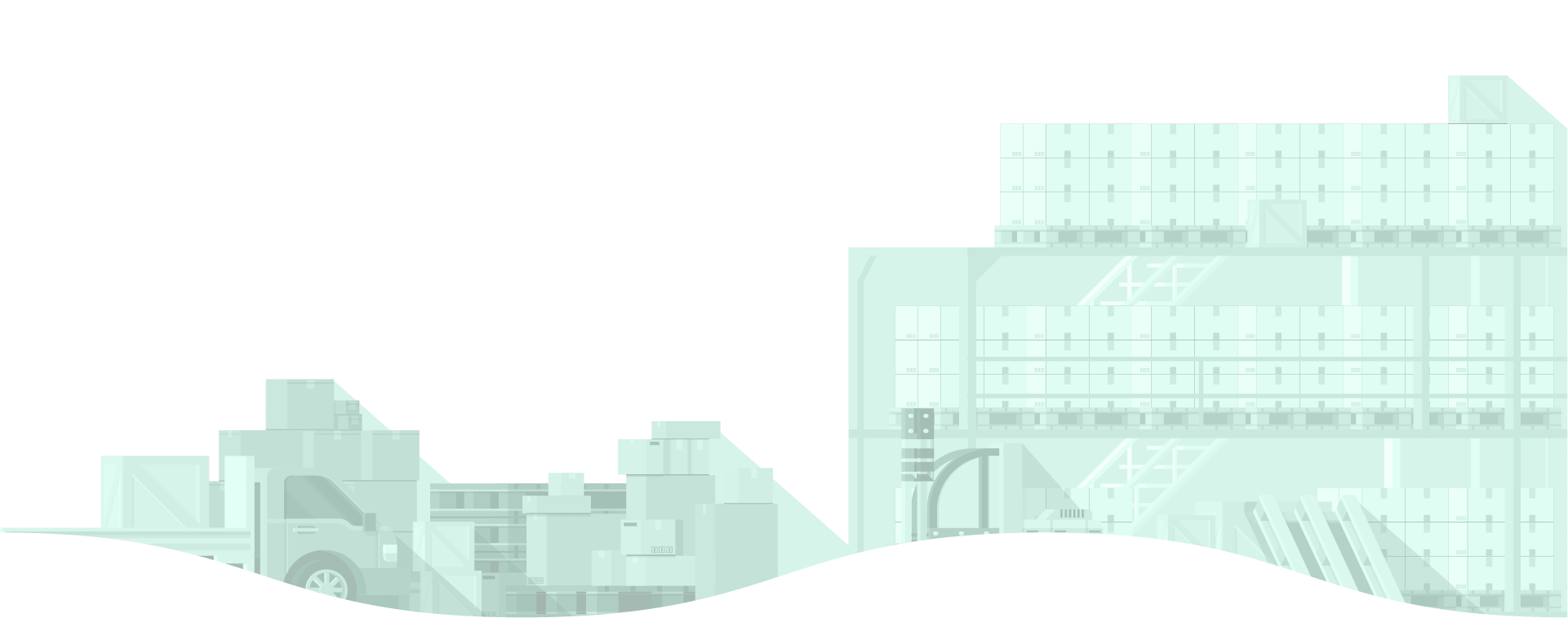corporate sector

2019

Total Assets & Equity
2015-2019

S\$ Bil

GROWTH RATE FROM 2018 TO 2019
TOTAL ASSETS
3.8%
TOTAL EQUITY
0.4%
COMPOSITION OF BALANCE SHEET
2019

Total Assets

s\$9338.2BIL

Total Equity

s\$3450.5BIL
of assets funded by equity

Total Liabilities

s\$5887.7BIL
TOTAL ASSETS BY INDUSTRY
2019TOTAL EQUITY BY TOP INDUSTRY
2019
CURRENT RATIO BY INDUSTRY
2019

Current assets < Current liabillities

Current assets = Current liabillities

Current assets > Current liabillities

1
RATIO BELOW 1
The risk of the company running into a liquidity problem is higher
RATIO EQUALS 1
The company has exactly balanced its current liabilities with current assets
RATIO ABOVE 1
This indicates liquidity in the company
Defined as the ratio of current assets to current liabilities and measures the liquidity of companies
EQUITY RATIO BY INDUSTRY
2019
The higher the company's dependence on external funding, the lower the ratio.
Defined as the ratio of total equity to total assets and measures the dependence of companies on external funding
RETURN ON ASSETS BY INDUSTRY
2019
Return on assets is defined as
the ratio of profit before interest and tax to average total assets.
RETURN ON EQUITY BY INDUSTRY
2019
Return on equity is defined as the ratio of profit before tax to average total equity.